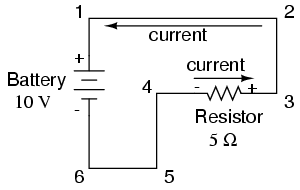# Polarity of voltage

We can trace the direction that electrons will flow in the same circuit by starting at the negative (-) terminal and following through to the positive (+) terminal of the battery, the only source of voltage in the circuit. From this we can see that the electrons are moving counter-clockwise, from point 6 to 5 to 4 to 3 to 2 to 1 and back to 6 again.

As the current encounters the 5 Ω resistance, voltage is dropped across the resistor’s ends. The polarity of this voltage drop is negative (-) at point 4 with respect to positive (+) at point 3. We can mark the polarity of the resistor’s voltage drop with these negative and positive symbols, in accordance with the direction of current (whichever end of the resistor the current is entering is negative with respect to the end of the resistor it is exiting:We could make our table of voltages a little more complete by marking the polarity of the voltage for each pair of points in this circuit:

Between points 1 (+) and 4 (-) = 10 volts
Between points 2 (+) and 4 (-) = 10 volts
Between points 3 (+) and 4 (-) = 10 volts
Between points 1 (+) and 5 (-) = 10 volts
Between points 2 (+) and 5 (-) = 10 volts
Between points 3 (+) and 5 (-) = 10 volts
Between points 1 (+) and 6 (-) = 10 volts
Between points 2 (+) and 6 (-) = 10 volts
Between points 3 (+) and 6 (-) = 10 volts

While it might seem a little silly to document polarity of voltage drop in this circuit, it is an important concept to master. It will be critically important in the analysis of more complex circuits involving multiple resistors and/or batteries.

It should be understood that polarity has nothing to do with Ohm’s Law: there will never be negative voltages, currents, or resistance entered into any Ohm’s Law equations! There are other mathematical principles of electricity that do take polarity into account through the use of signs (+ or -), but not Ohm’s Law.

• REVIEW:
• The polarity of the voltage drop across any resistive component is determined by the direction of electron flow though it: negative entering, and positive exiting.

COMMENT songs